• Matlab--使用HeatMap画热图
注意：HeatMap和heatmap是不一样的！
使用HeatMap画热图：
clc;
clear;
data = [0,40,0,31,0,0,0,0;
30,0,40,0,2,0,1,0;
0,0,0,0,0,13,0,60;
39,0,31,0,1,0,0,0;
0,30,0,39,0,1,0,0;
1,0,1,0,56,0,12,0;
0,2,0,1,0,56,0,12;
0,0,0,0,12,0,59,0];
xvalues = {'CNC1','CNC2','CNC3','CNC4','CNC5','CNC6','CNC7','CNC8'};
yvalues = {'CNC1','CNC2','CNC3','CNC4','CNC5','CNC6','CNC7','CNC8'};
yvalues=yvalues';
h=HeatMap(data,'Colormap',redbluecmap,'ColumnLabels',yvalues,'RowLabels',xvalues);
h.Annotate = true;%在热图中显示数据值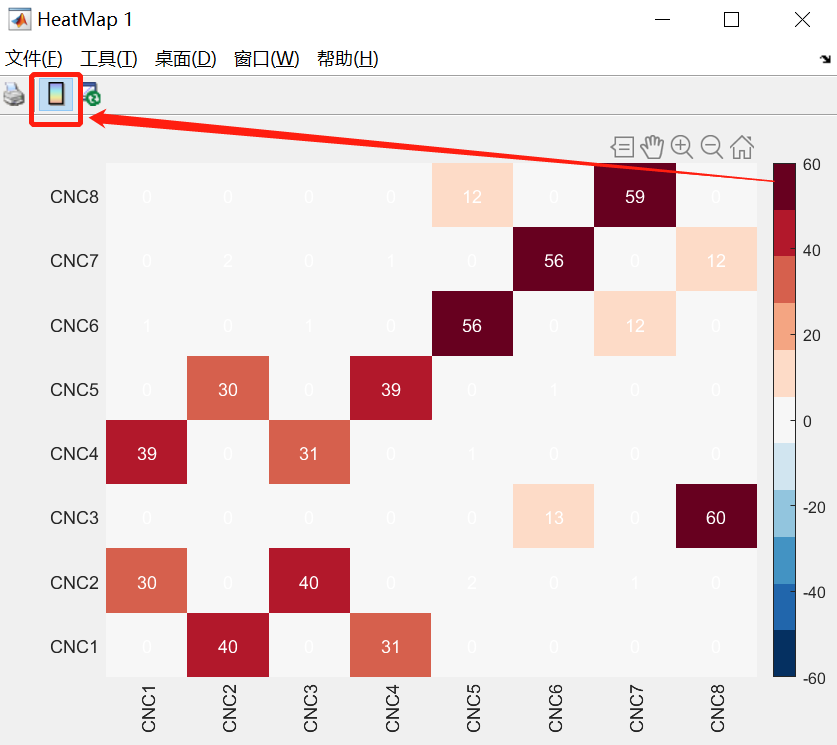颜色条可以使用GUI的界面插入colorbar
展开全文• 网上找到的画热图的方法大部分跟我预期都有差距，最后找到了一个代码，然后经过改良可以用来画热图/分布图。 这个代码主要是用到通过hist3这个函数对数据进行分类，然后用scatter函数进行绘制。 以随机生成x和y成...
网上找到的画热图的方法大部分跟我预期都有差距，最后找到了一个代码，然后经过改良可以用来画热图/分布图。
这个代码主要是用到通过hist3这个函数对数据进行分类，然后用scatter函数进行绘制。
以随机生成x和y成正态分布均值为0，方差为10的图为例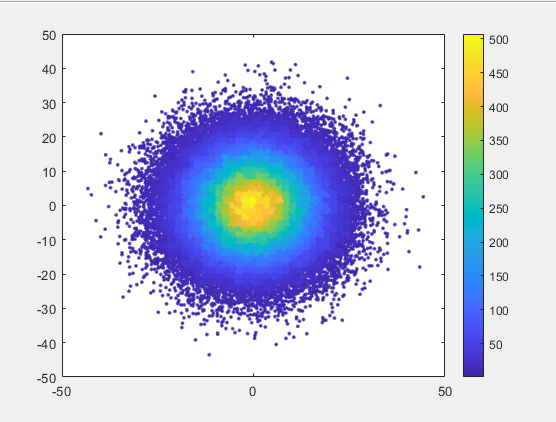X=normrnd(0,10,[100000,1]);
Y=normrnd(0,10,[100000,1]);
numbins = 50;
markersize = 50;
marker = '.';
[values, centers] = hist3([X Y], [numbins numbins]);
centers_X = centers{1,1};
centers_Y = centers{1,2};
binsize_X = abs(centers_X(2) - centers_X(1)) / 2;
binsize_Y = abs(centers_Y(2) - centers_Y(1)) / 2;
bins_X = zeros(numbins, 2);
bins_Y = zeros(numbins, 2);
for i = 1:numbins
bins_X(i, 1) = centers_X(i) - binsize_X;
bins_X(i, 2) = centers_X(i) + binsize_X;
bins_Y(i, 1) = centers_Y(i) - binsize_Y;
bins_Y(i, 2) = centers_Y(i) + binsize_Y;
end
scatter_COL = zeros(length(X), 1);
onepercent = round(length(X) / 100);
for i = 1:length(X)
last_lower_X = NaN;
last_higher_X = NaN;
id_X = NaN;
c_X = X(i);
last_lower_X = find(c_X >= bins_X(:,1));
if (~isempty(last_lower_X))
last_lower_X = last_lower_X(end);
else
last_higher_X = find(c_X <= bins_X(:,2));
if (~isempty(last_higher_X))
last_higher_X = last_higher_X(1);
end
end
if (~isnan(last_lower_X))
id_X = last_lower_X;
else
if (~isnan(last_higher_X))
id_X = last_higher_X;
end
end
last_lower_Y = NaN;
last_higher_Y = NaN;
id_Y = NaN;
c_Y = Y(i);
last_lower_Y = find(c_Y >= bins_Y(:,1));
if (~isempty(last_lower_Y))
last_lower_Y = last_lower_Y(end);
else
last_higher_Y = find(c_Y <= bins_Y(:,2));
if (~isempty(last_higher_Y))
last_higher_Y = last_higher_Y(1);
end
end
if (~isnan(last_lower_Y))
id_Y = last_lower_Y;
else
if (~isnan(last_higher_Y))
id_Y = last_higher_Y;
end
end
scatter_COL(i) = values(id_X, id_Y);
end
scatter(X, Y, markersize, scatter_COL, marker);
hold on
colorbar;
box on

展开全文• 为值在 Z 中的 (X,Y) 坐标绘制二维热图X, Y , Z 必须是列创建人：Eng。 Osama Talaat Abdel-Hafiz - 博士生埃及 - 2017 年 9 月
• 如图所示，有的时候叫热图(可能根据不同的色彩分别吧)，下面简单介绍下，有点累人的图。(Matlab所作，后面会给出代码) 具体需要看这幅图的横坐标与纵坐标的粒度是多少，比如这幅图，每幅子图选取的粒度为0.025，...
如图所示，有的时候叫热图(可能根据不同的色彩分别吧)，下面简单介绍下，有点累人的图。(Matlab所作，后面会给出代码)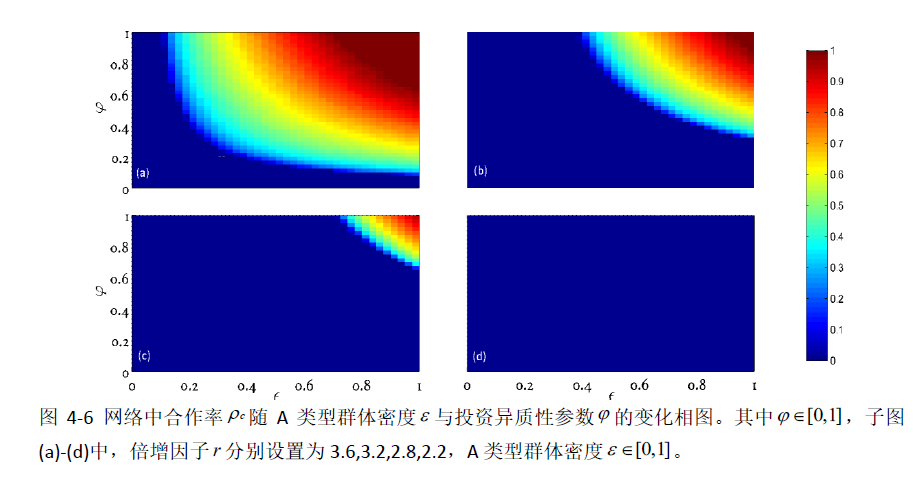具体需要看这幅图的横坐标与纵坐标的粒度是多少，比如这幅图，每幅子图选取的粒度为0.025，所以横坐标与纵坐标都需要一致(都为0.025，至少我做的是这样，如有问题请指出)，其实每个点都是一个像素，比如说(0.025,0.025)就是一个像素，每个像素都是一个数值

得到的部分数据格式如下：

第一列为横坐标，第二列为纵坐标，第三列为像素点(代表你所需要的指标，在这幅图中代表群体合作率)

前端：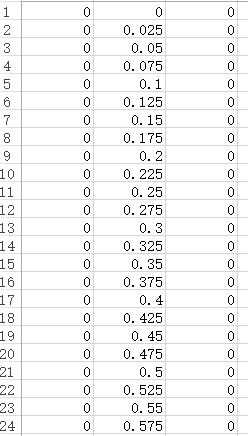后端：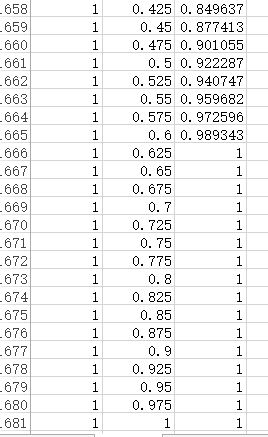由数据格式可知，这是一个全集，即每个点都有另外的点会有结果，所以一共有1600个结果，每幅子图都是由1600个结果画成的。

matlab代码：

subplot(2,2,1,'Position',[0.15 0.56 0.3 0.4])
[X,Y] = meshgrid(0:0.025:1.0,0:0.025:1.0);
Z = reshape(num,41,41);
surf(X,Y,Z);

ylabel('$$\varphi$$','interpreter','laTex');

set(gca,'fontsize',10,'FontName','High Tower Text','box','on','xtick',[],'ytick',[0:0.2:1.0]);grid on

subplot(2,2,2,'Position',[0.50 0.56 0.3 0.4])
[X,Y] = meshgrid(0:0.025:1.0,0:0.025:1.0);
Z = reshape(num,41,41);
surf(X,Y,Z);

axis off
grid on

subplot(2,2,3,'Position',[0.15  0.09 0.3 0.4])

[X,Y] = meshgrid(0:0.025:1.0,0:0.025:1.0);
Z = reshape(num,41,41);
surf(X,Y,Z);

xlabel('$$\epsilon$$','interpreter','LaTex');
ylabel('$$\varphi$$','interpreter','laTex');

set(gca,'fontsize',10,'FontName','High Tower Text','box','on','xtick',[0:0.2:1.0],'ytick',[0:0.2:1.0]);grid on
subplot(2,2,4,'Position',[0.50  0.09 0.3 0.4])
[X,Y] = meshgrid(0:0.025:1.0,0:0.025:1.0);
Z = reshape(num,41,41);
surf(X,Y,Z);
xlabel('$$\epsilon$$','interpreter','LaTex');

set(gca,'fontsize',10,'FontName','High Tower Text','box','on','xtick',[0:0.2:1.0],'ytick',[]);
grid on

代码画的是四幅图，可能会涉及调节位置的问题。
有的时候，图形导出来会有类似白色条形状，可以用高清图导出试试，代码如下：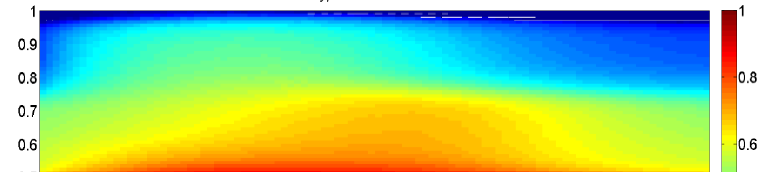set(gcf, 'PaperPositionMode', 'auto')
print -dpng -r1700 f3.png
展开全文heatmap
• %原距离图的垂直镜像,本身是反的，否则出来是反的，用flipdim函数 h=pcolor(depth2);%热度图 axis equal set(h,‘edgecolor’,‘none’,‘facecolor’,‘interp’);%去掉网格，平滑热度图 colorbar;%显示图例 ...
热力图主要用pcolor函数， img=imread(‘E:\Data_Set\RESIDE\OTS_BETA（室外合成）\clear\clear\0138.jpg’) ; load(‘E:\Data_Set\RESIDE\OTS_BETA（室外合成）\depth\depth\0138.mat’) subplot(1,2,1) depth2=flipdim(depth,1);%原距离图的垂直镜像,本身是反的，否则画出来是反的，用flipdim函数 h=pcolor(depth2);%热度图 axis equal set(h,‘edgecolor’,‘none’,‘facecolor’,‘interp’);%去掉网格，平滑热度图 colorbar;%显示图例 subplot(1,2,2) imshow(img)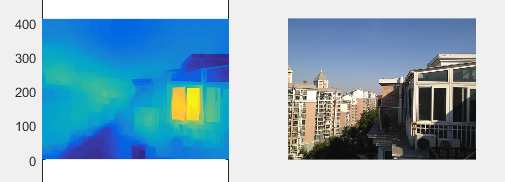do_show_log_scale=1;
max_d=1.9134;
min_d=-0.0315;
max_d=power(10, max_d);
min_d=power(10, min_d);

if do_show_log_scale
scaling_label=log10(max_d)-log10(min_d);
offset_label=log10(min_d);
else
scaling_label=max_d-min_d;
offset_label=min_d;
end

depth_inpaint_show=data_obj;
if do_show_log_scale
depth_inpaint_show=log10(data_obj);
end
depth_inpaint_show=(depth_inpaint_show - offset_label)/scaling_label;

% one_cache_file='predict_depth_gray.png';
subplot(1,2,1)
imshow(depth_inpaint_show)

% one_cache_file='predict_depth_rgb.png';
depth_show=depth_inpaint_show;
depth_show=(depth_show-min(depth_show(:)))/(max(depth_show(:)) - min(depth_show(:)));
depth_show=depth_show*(64-1)+1;
depth_show=round(depth_show);
subplot(1,2,2)
imshow(depth_show, colormap('jet'))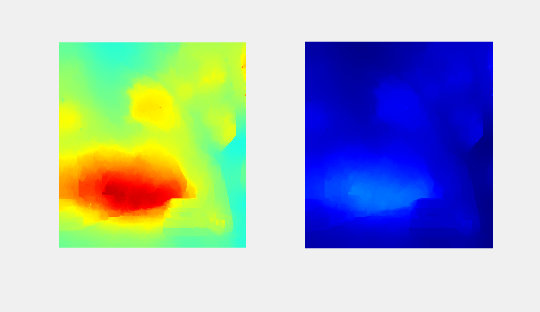ref https://blog.csdn.net/Eric_KEY/article/details/64602292 https://www.cnblogs.com/chenwenyan/p/6836578.html https://blog.csdn.net/liuyifang0810680/article/details/78784102
展开全文• ## matlab绘制热力图

千次阅读 2021-05-07 23:06:03
data2=[0.617234569 0.617234569 4.320975309 0.617308642 4.320975309; 8.024567902 0.617234569 2.46908642 1.851814816 0.617308642; 0.617234569 1.851814816 0 0 1.851814816; 0.617308642 0.617308642 1....
• ## matlab画热力图

万次阅读 2019-09-01 18:42:10热力图 imagesc
• 允许在圆形热图绘制数据数组的功能。 可选地，可以通过使用单元阵列以及每个单元的每个组的数据阵列来实现空间上分离的组。 允许在文件交换上输入cmocean工具箱颜色图。 例子： 关闭所有清除 %%在线示例 DataX {1...
• matlab平台实现，功能为生成热力散点图untitled.m为运行代码
• figure,imshow(input);colormap(jet); f=getframe(gcf); imwrite(f.cdata,[‘output’,’.png’]) 欢迎使用Markdown编辑器 你好！ 这是你第一次使用 Markdown编辑器 所展示的欢迎页。如果你想学习如何使用Markdown...
• ## matlab画热力网格图

千次阅读 多人点赞 2020-01-12 23:24:39
Matlab做热力图 内容 环境配置 加载数据以进行可视化 简单的热图】 轴刻度和标签 热图文字标签 缩放，平移和数据光标 更改颜色图 图上有多个热图 强制色阶 缺失值 彩条 网格线 1 配置环境 将...热力图
• https://blog.csdn.net/sinat_17306241/article/details/102732746MATLAB画图颜色渐变 Matlab 绘制三维散点图并进行曲面拟合
• polarPcolor旨在用极坐标表示极坐标中的伪彩色图，并提供径向网格以使数据清晰可见。 例如，它非常适合雷达或激光雷达的平面位置指示...  http://www.mathworks.com/matlabcentral/fileexchange/13200-3d-polar-plot
• 如果% 你想用 0.5-0.8 的范围绘制 Y 轴（以显示更窄的% FRET 范围），然后使用 0.5、0.8。 ％[**] 50是要在X轴上显示的帧数。 如果你% 希望在 .dat 文件中显示前 50 个数据点（如下所述）。然后使用 50。 % [**] ...
• 绘制FRET效率值随时间变化的2D热图。 .m文件（RETStatesDistribution_withTime_ByOverlaying.m）存在于附加的.zip文件夹中。 它还包含一些示例数据（.dat文件），可用于对其进行测试。 请随时使用。 没有版权或使用...
• 文章目录 cdata = [1 2 3 4 5; 5 4 3 2 1; 1 2 3 4 5; 5 4 3 2 1; 1 2 3 4 5]; xvalues = {'1x', '2x', '3x', '4x... Ref: 创建热图- MATLAB heatmap - MathWorks 中国 看完这篇，还有你不会的热力图吗? matlab热力图
• reference:https://jingyan.baidu.com/article/870c6fc36fdacfb03ee4be58.html ... MATLAB是著名的科学软件，具有绘图、编程、仿真等强大的功能。现在介绍MATLAB绘制中国地图的三种方式，分别是（1）使用m_...
• 如果已经直到第一维和第二维代表的意义, 就可以直接该热度图了. 假设该矩阵为A, 第一维是f, 第二维为t 用mesh命令 [X,Y] = meshgrid(t,f); %设定每个元素的坐标 mesh(X,Y,A) % 画图 xlim([t(1),t(end)]) % 去掉...
• 主要实现以下功能： 根据不同的工作簿读取xlsx数据 根据给定矩阵，绘画热力图 更改热力图的颜色模式 按照指定步长设置x轴和y轴的刻度 根据x轴和y轴重命名刻度值 使用text（）函数来，给图像指定位置添加标注 ...
• 超宽带四个基站，设置25个测试点，出测距误差分布热图（含数据），matlab
• 转换原理为原图像像素值越大，在热图中就越红，反之在热图中就越蓝。 colormap(jet);使用的jet色图如下： 代码： 结果： 原reference：https://blog.csdn.net/zhangeximu/article/details/89496212 ...
• 使用matlab软件处理脑电信号处理eeg。使用morlet小波进行小波变换，并出它的时间频率能量图。
• Matlab绘制六边形蜂窝网路并编号目录用 [TOC]来生成目录：Matlab绘制六边形蜂窝网路并编号目录 代码块 效果展示 核心说明 单输入值控制颜色变换代码块clear;clc rc=4;dy=2*rc;dx=rc*sqrt(3); A=pi/3*[1:7]; rol=50; ...
• 该函数设置了用于创建不同六边形片段的热图的体系结构，这些热图总体上创建了一个巨型六边形。
• ## 画相关性热图

千次阅读 2019-06-12 16:24:00
数据格式如下 Sample CM11 CM12 CM13 CM21 CM22 CM23 CM31 CM32 CM33 CM11 1 0.9627369 0.9884578 0.9841946 0.9762492 0.983613 0.9575127 0.743262 0.6178 CM12 0.9627369 1 0.9616447 0.9405868 0.9354329...
• matlab绘制曲线代码离体钙成像数据处理 Matlab 代码是用于图像加载（使用内存映射）、ROI 绘制和 dF/F 计算的定制 GUI R 脚本用于处理来自 MATLAB GUI 的 ROI 读数。 其中包括制作dF/F曲线、统计分析、热图等。
• 直白说明： xlabel('{\itx}/m') 出现的结果： x/m 也就是说： {\itx}将x斜体化了~ 明白了吧~xlabel...

# matlab画热图matlab 订阅